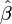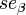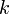# T-Test of a Regression Coefficient

Where$\hat{\beta}$ is a coefficient,$n$ is the effective number of observations (note that this is different to the effective sample size where repeated observations exist for each respondent) and$se_{\beta}$ is the standard error of the coefficient, the test statistic is:$t=\frac{\hat{\beta}}{se_{\beta}}$,$p = 2\Pr(t_{n-k} \ge |t|)$,$k$ is the number of parameters, including the intercept (where applicable).